## 13.5.2 Differential Game Theory

The extension of sequential game theory to the continuous-time case is called differential game theory (or dynamic game theory ), a subject introduced by Isaacs . All of the variants considered in Sections 9.3, 9.4, 10.5 are possible:

1. There may be any number of players.
2. The game may be zero-sum or nonzero-sum.
3. The state may or may not be known. If the state is unknown, then interesting I-spaces arise, similar to those of Section 11.7.
4. Nature can interfere with the game.
5. Different equilibrium concepts, such as saddle points and Nash equilibria, can be defined.
See  for a thorough overview of differential games. Two players,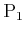and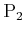, can be engaged in a differential game in which each has a continuous set of actions. Let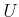and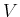denote the action spaces ofand, respectively. A state transition equation can be defined as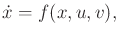(13.203)

in whichis the state,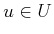, and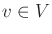.

Linear differential games are an important family of games because many techniques from optimal control theory can be extended to solve them .

Example 13..17 (Linear Differential Games)   The linear system model (13.37) can be extended to incorporate two players. Let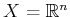be a phase space. Let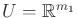and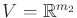be an action spaces for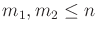. A linear differential game is expressed as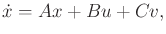(13.204)

in which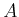,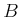, and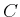are constant, real-valued matrices of dimensions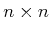,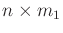, and, respectively. The particular solution to such games depends on the cost functional and desired equilibrium concept. For the case of a quadratic cost, closed-form solutions exist. These extend techniques that are developed for linear systems with one decision maker; see Section 15.2.2 and .

The original work of Isaacs  contains many interesting examples of pursuit-evasion differential games. One of the most famous is described next.

Example 13..18 (Homicidal Chauffeur)   In the homicidal chauffeur game, the pursuer is a Dubins car and the evader is a point robot that can translate in any direction. Both exist in the same world,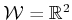. The speeds of the car and robot are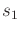and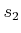, respectively. It is assumed that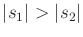, which means that the pursuer moves faster than the evader. The transition equation is given by extending (13.15) to include two state variables that account for the robot position: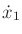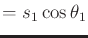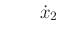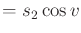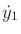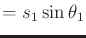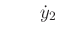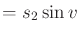(13.205)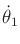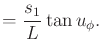The state space isis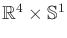, and the action spaces are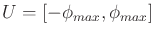and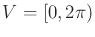.

The task is to determine whether the pursuer can come within some prescribed distance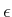of the evader: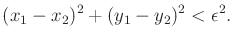(13.206)

If this occurs, then the pursuer wins; otherwise, the evader wins. The solution depends on the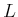,,,, and the initial state. Even though the pursuer moves faster, the evader may escape because it does not have a limited turning radius. For given values of,,, and, the state spacecan be partitioned into two regions that correspond to whether the pursuer or evader wins [59,477]. To gain some intuition about how this partition may appear, imagine the motions that a bullfighter must make to avoid a fast, charging bull (yes, bulls behave very much like a fast Dubins car when provoked).Another interesting pursuit-evasion game arises in the case of one car attempting to intercept another .

Example 13..19 (A Game of Two Cars)   Imagine that there are two simple cars that move in the same world,. Each has a transition equation given by (13.15). The state transition equation for the game is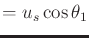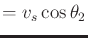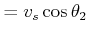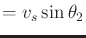(13.207)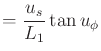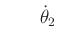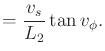The pursuit-evasion game becomes very interesting if both players are restricted to be Dubins cars.Steven M LaValle 2020-08-14